Next:Dimensional Unified Divergence MeasuresUp:Dimensional Divergence Measures

M-Dimensional Divergence Measures

Let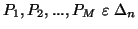be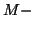probability distributions. Let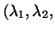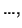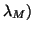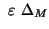. It is well known that the Shannon's entropy satisfy the following inequality: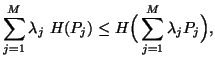(5.1)

with equality iff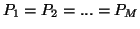.

Also due to property 1.14, we have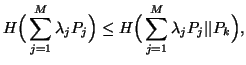(5.2)

for each k=1,2,...,M with equality iff.

We can easily check that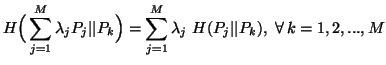(5.3)

Multiplying both sides of (5.2) by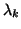, summing over k=1,2,...,M and using (5.3), we have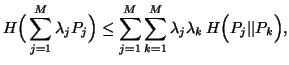(5.4)

with equality iff.

From (5.1) and (5.4), we have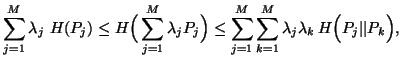(5.5)

with equality iff.

The above inequality (5.5) admits the following three nonnegative differences given by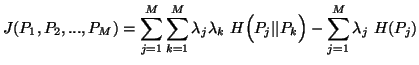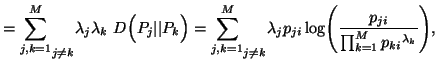(5.6)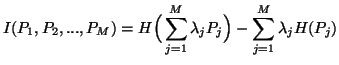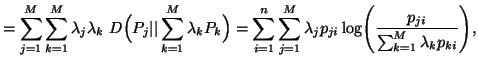(5.7)

and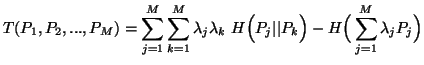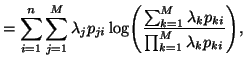(5.8)

From (5.6), (5.7) and (5.8), we conclude that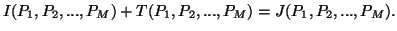(5.9)

From (5.9), we have the following inequalities: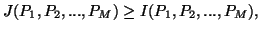(5.10)

and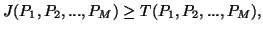(5.11)

The measures (5.6), (5.7) and (5.8) in particular reduce to (2.6) and (2.7) respectively, when M=2,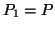and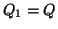with a multiplicative constant.

Note 5.1.

(i) The measure (5.6) is adimensional generalization of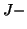divergence and is studied for the first time by Toussaint (1978) . The measure (5.7) isdimensional generalization of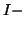divergence (2.8) and is studied for the first time by Burbea and Rao (1982a) . While the measure (5.8) is new. It invloves the expressions appearing in the arithmetic and geometric mean inequality given by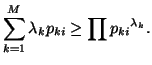(ii) We call the measure (5.8) the arithmetic and geometric mean divergence measure or simply a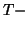divergence. It has been studied by Taneja (1995) .

21-06-2001
Inder Jeet Taneja
Departamento de Matemática - UFSC
88.040-900 Florianópolis, SC - Brazil